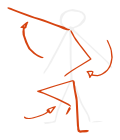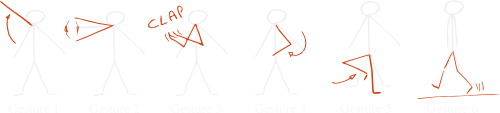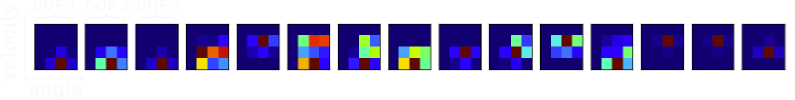## Learning to recognize parallel motion primitives with linguistic descriptions using NMF

### Introduction

We study the following question: How to learn simple gestures from the observation of choreographies that are combinations of these gestures? We believe that this question is of scientific interest for two main reasons.

#### Choreographies are combinations of parallel primitive gesturesNatural human motions are highly combinatorial in many ways. Sequences of gestures such as walk to the fridge; open the door; grab a bottle; open it; ...'' are a good example of the combination of simple gestures, also called primitive motions, into a complex activity. Being able to discover the parts of a complex activity and learn them, reproduce them and compose them into new activities by observation is thus an important step towards imitation learning or human behavior understanding for capability for robots and intelligent systems.

A substantial amount of research has been done towards the learning of parts of motions combined into sequences. In an orthogonal direction, we are interested in primitive motions that are combined together at the same time. We denote this kind of combinations as parallel combinations. A dancer executing a choreography provides a prototypical example of such a combination. Indeed a choreography might be formed by executing some particular step, a gesture with the left arm while maintaining some posture of the head and the right arm at the same time.

#### Relation between motion and language learning

The general problem of learning a dictionary of primitive gestures which combinations can explain the observed set of choreographies is generally ill-posed. Actually several (sometime an infinity of) dictionaries could be chosen. Thus some heuristic, other than the recurring patterns in the signal, have to be selected to guide the choices and remove ambiguities. Examples of such heuristic are given below:

• Structural cues: some solutions are more appealing because they are simpler in some way (e.g. sparse representation, easier to reproduce, ...),
• Developmental cues: some solutions are more appealing because they better re-use previous knowledge (a Chinese child may not remember a new sound the same way a Spanish child would),
• Social and multimodal cues: the learner is guided towards a certain solution by a caregiver or because it is more coherent with what it sees, hears, touch, etc.

This work focuses on the last element, that is to say social cues. More precisely we are interested in the case where examples of motions presented during learning are associated with linguistic descriptions.

Interestingly, the combination of phonemes into meaningful words and sentences in language presents properties similar to the combination of motor primitives into complex behaviors. As an instance of combined motor and language learning, this problem illustrated a situation where language can shape or even improve the learning of motor skills for example. On the other hand, such combined learning provides a plausible answer to the symbol grounding problem.

In , Driesen et al. explore a similar problem where part of spoken language (words) are learnt autonomously from spoken utterances associated to scenes they described. In their work the scenes are described by sets of symbolic labels that could also describe the choreographies that we study. Our goal is thus to develop techniques that could learn from both spoken utterance and real demonstrations of choreographies. We achieved a first step toward this goal by performing the symmetric experiment where parts of real dance motions are learnt through the observation of the choreographies and a symbolic linguistic description. Because we adapted the algorithm from Driesen et al. to perform this experiment it demonstrates a similarity between language and motion learning.

### Matrix factorization for multi-modal learning

Matrix factorization is a class of techniques that can be used in machine learning to solve dictionary learning problems similar to the one we are interested in. We assume that the samples $$v^i$$ that are observed can be expressed as the linear combination of atoms $$w^j$$ from the dictionary: $v^i = \sum\limits_{j=1}^{k}h_i^jw^j$ The set of samples are represented by a matrix $$V$$, the set of atoms by a matrix $$W$$ and the coefficients by a matrix $$H$$. The previous equation can thus be re-written as: $V \simeq W\cdot H$ The equality from the formal model has been replaced by the approximation that is optimized in practice. Finding the matrices $$W$$ and $$H$$ allows good approximation is thus a way of learning a decomposition of the observed data into recurrent parts.

In order to apply the matrix factorization techniques to the learning in a multimodal setup, Driesen et al. have proposed the following approach. We suppose that each observation $$v$$ is composed of a part representing the motion and a part representing the linguistic description. A language part and a motion part can also be identified in the data matrix $$V$$ and the dictionary matrix $$W$$. The coefficients from $$H$$ are an internal combined representation of the data and thus do not contain a separate language and motion part.Then the idea is to use the multimodal training data to learn the dictionary. Once the dictionary is learnt, if only the motion data is observed, the motion part of the dictionary can be used to infer the internal coefficients. The internal coefficients can be used afterward to reconstruct the linguistic part associated with the observed motion. A similar process enables the reconstruction of the motion representation associated to an observed linguistic description.We more specifically use non-negative matrix factorization (NMF, , ) which requires that we represent motion by vectors with non-negative values. Such a representation is detailed below.

### Motion capture

We have recorded a dataset of real human dance motions recorded through a Microsoft Kinect™ device. This dataset is publicly available at http://flowers.inria.fr/choreography_database.html along with its documentation.### Motion representation

We use a motion representation based on histograms of position velocity. From the sequence of angular position of each joint of the body, we consider each joint separately and computes the angular velocity of the joint with respect to time. Then we compute a 2D histogram from the couples of angular positions and angular velocities. Finally we flatten the 2D histogram corresponding to each joint and concatenate the histograms from all joints into a vector of non-negative values representing the motion.### Results

In our IROS 2012 paper we experimented with this approach on data from the choreography dataset. The results show that the system is capable of learning a multimodal dictionary that enables it to later reproduce the linguistic demonstration associated with an unknown demonstrated choreography.

### Bibliography

1. Driesen J., ten Bosch L., Van Hamme H., Adaptive Non-negative Matrix Factorization in a Computational Model of Language Acquisition. Interspeech, 2009
2. Paatero P., Tapper U., Positive matrix factorization: A non-negative factor model with optimal utilization of error estimates of data values. Environmetrics, 1994
3. Lee D. D., Seung H. S., Learning the parts of objects by non-negative matrix factorization. Nature, 1999